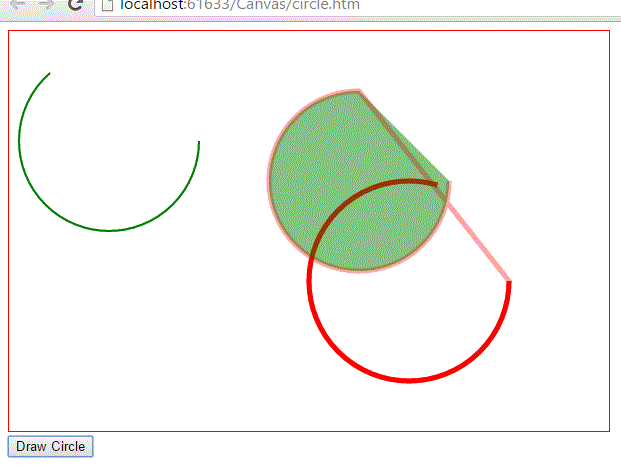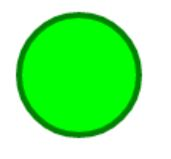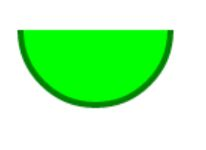# Canvas Circle in HTML5

##### How to draw a circle on canvas in HTML5?

By using the function `Drawcircle()` in `<script>` code we can draw the circle on canvas.

```<script>
// to more about arc, visit http://simeonvisser.hubpages.com/hub/HTML5-Tutorial-Drawing-Circles-and-Arcs
function DrawCircle() {
var context = document.getElementById("myCanvas").getContext("2d");

// context.beginPath();
context.lineWidth = "5";
// arc(x center coordinate, y center coordinate, radius, start angle value, end angle value, clock wise/anti clock wise=true)
// start and end angles are measured in radius. 360 degree = Mapth.PI * 2
context.arc(400, 250, 100, 0, 5, false);
context.strokeStyle = "red";
context.stroke(); // mandatory to call

context.beginPath();
context.lineWidth = "2";
context.strokeStyle = "green";
context.arc(100, 110, 90, 0, 4, false);

context.stroke(); // mandatory to call again

context.beginPath();
context.arc(350, 150, 90, 0, Math.PI * 1.5, false);
context.fillStyle = "green";
context.globalAlpha = 0.2;
context.fill();

}
</script>

<body>
<canvas id="myCanvas" width="600" height="400" style="border: 1px solid red;">Canvas is not supported in your browser
</canvas>
<br />
<input type="button" onclick="DrawCircle()" value="Draw Circle" />
</body>
```

In the above code snippet we have given getElementById as the `<canvas>` Id and there are six parameters used in the code. They are as follows

• 1st parameter X center co-ordinate, where we need to keep the origin of the circle.
• 2nd parameter Y center co-ordinate, where we need to keep the origin of the circle (As we know the origin of the circle is [0, 0] i.e X co-ordinate center is 0 and Y Co-ordinate center is 0).
• 3rd parameter is radius, with how much radius we need to draw the circle. Radius is nothing but the half of the diameter.
• 4th parameter is start angle value, with how much angle we need to start  drawing the circle.
• 5th parameter is end angle value, with how much angle we need to end drawing the circle.
• 6th parameter is Clock wise/ Anti clock wise, indicates about the direction of drawing the circle on canvas.

Below the `<canvas>` element there is an `<input>` element which consists of "Button". In the output you can observe empty canvas area and below that button with value "Draw Circle" initially. By clicking on the button you can observe the formation of circles inside the canvas area as per the given input values.

OUTPUT### Circles

These are used to create circles

``` <canvas id="myCanvas" width="600" height="400"></canvas>
<script>
var canvas = document.getElementById('myCanvas');
var context = canvas.getContext('2d');
var centerX = canvas.width / 6;
var centerY = canvas.height / 4;

context.beginPath();
context.arc(centerX, centerY, radius, 0, 2 * Math.PI, false);
context.fillStyle = 'lime';
context.fill();
context.lineWidth = 4;
context.strokeStyle = 'green';
context.stroke();
</script>
```

Circle

In the above code snippet we have defined the circles

we have defined id as "mycanvas"

• var centerX = canvas.width / 6;= it divides the width by 6
• var centerY = canvas.height / 4;- it divides rthe height by 4
• context.arc(centerX, centerY, radius, 0, 2 * Math.PI, false);= it defines the arc of the circ;le of the image
• context.fillStyle = 'lime';= it defines the color as lime
• context.lineWidth = 4;= it defines the line width as 4
• context.strokeStyle = 'green';= it defines the color of the line widthas green

output### Semicircle

In order to create the semicircle we need to follow this approach

```<canvas id="myCanvas" width="600" height="400"></canvas>
<script>
var canvas = document.getElementById('myCanvas');
var context = canvas.getContext('2d');
var centerX = canvas.width / 8;
var centerY = canvas.height / 4;

context.beginPath();
context.arc(centerX, centerY, radius, 0, Math.PI, false);
context.fillStyle = 'lime';
context.fill();
context.lineWidth = 4;
context.strokeStyle = 'green';
context.stroke();
</script>
```

Semicircle

In the above code snippet we have created the semicircle. we need to add the math.pi to create the semicircle

• var centerX = canvas.width / 6;= it divides the width by 6
• var centerY = canvas.height / 4;- it divides rthe height by 4
• context.arc(centerX, centerY, radius, 0, Math.PI, false);= it defines the arc of the circ;le of the image
• Math.pi is used vto create the semicircle
• context.fillStyle = 'lime';= it defines the color as lime
• context.lineWidth = 4;= it defines the line width as 4
• context.strokeStyle = 'green';= it defines the color of the line widthas green

outputViews: 5677 | Post Order: 43